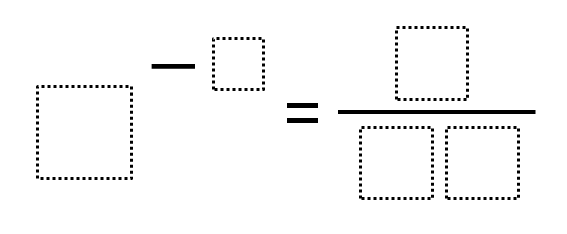# Negative Exponents

Directions: Using the digits 0 to 9, at most one time each, place a digit in each box to make a true statement.### Hint

How does a negative exponent result in a fractional solution?
How do we know where the 1 should go?

There are multiple answers such as:

3^ (-2) = 1 / 09
7 ^ (-2) = 1 / 49
8 ^ (-2) = 1 / 64
2 ^ (-3) = 1 / 08

Source: Daniel Luevanos

## Exponential Powers

Directions: Using the digits 0 to 9 at most one time each, place a digit …

1.3^-2=6/54

2.Good spot Joe! 🙂

Using your appraoch I also found 3^-2 = 9/81

3.there are 43 solutions

4.7^-2 = 1/49